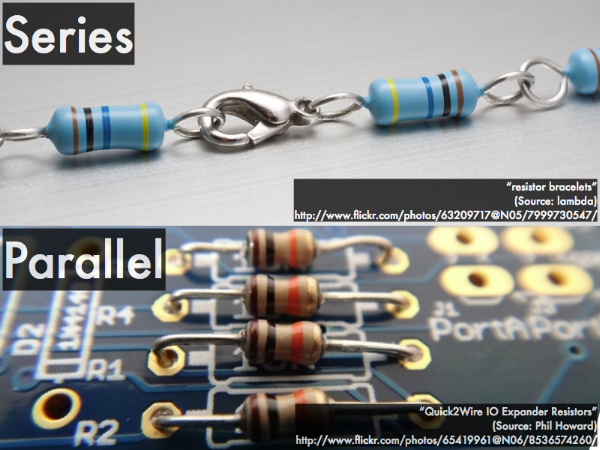## 20160318

### Online reading assignment: circuit analysis

Physics 205B, spring semester 2016
Cuesta College, San Luis Obispo, CA

Students have a bi-weekly online reading assignment (hosted by SurveyMonkey.com), where they answer questions based on reading their textbook, material covered in previous lectures, opinion questions, and/or asking (anonymous) questions or making (anonymous) comments. Full credit is given for completing the online reading assignment before next week's lecture, regardless if whether their answers are correct/incorrect. Selected results/questions/comments are addressed by the instructor at the start of the following lecture.

The following questions were asked on reading textbook chapters and previewing presentations on circuit analysis.Selected/edited responses are given below.

Describe what you understand from the assigned textbook reading or presentation preview. Your description (2-3 sentences) should specifically demonstrate your level of understanding.
"How a series circuit works now. They are easy to understand and I believe that I can solve problems involving them to an extent."

"Current in equals current out, whether in series or parallel."

"Not much was confusing, it was all explained in simple to understand terms. I understand some of the differences between series and parallel wiring, but will benefit from review on this topic."

"Resistances can be in series or in parallel. When in parallel, the sum of the resistances is the sum of the reciprocals' reciprocal (?). This means that the resistances become smaller when a circuit is in parallel! (Why?) If current flows from left to right then ∆V drops (dropping EPE per charge) and vice versa for right to left. Kirchhoff's rules explains how charge and energy are conserved throughout the circuit."

"Resistors can be configured into two ways. Resistors can be in a series, where the current flows through one resistor at a time on one path. The other configuration is the parallel series, which allows the current to pass through multiple resistors simultaneously."

Describe what you found confusing from the assigned textbook reading or presentation preview. Your description (2-3 sentences) should specifically identify the concept(s) that you do not understand.
"I am confused with potential drops and raises. Discussion of this would help a lot."

"How exactly the current splits in parallel wirings."

"I will benefit from class examples on the differences between series and parallel circuits."

"How is it that when resistors are in parallel they use the same amount of voltage but the current gets divided?"

"I don't understand Kirchhoff's rule or the loop concept, I thought I did but when it came to calculating the math portion of the problems I didn't understand how to complete the problem..."

"I do not understand about Kirchhoff's rules. I just do not get how to apply it."

"Everything!!!!"

Determine what happens to the following parameters as current flows through an ideal wire.
(Only correct responses shown.)
Current: remains the same [81%]
Voltage: remains the same [56%]Determine what happens to the following parameters if you go through a resistor along the direction of current.
(Only correct responses shown.)
Current: remains the same [66%]
Voltage: decreases [59%]Determine what happens to the following parameters if you go through into the (-) terminal and out of the (+) terminal of an ideal battery.
(Only correct responses shown.)
Current: remains the same [59%]
Voltage: increases [69%]

Briefly explain what quantity is conserved when applying Kirchhoff's junction rule.
"Not sure."

"Current."

"The current is conserved."

"Conservation of charge (i.e. current) is conserved."

Briefly explain what quantity is conserved when applying Kirchhoff's loop rule.
"Voltage."

"Electric potential energy per charge."

"Conservation of energy (per charge)."

"Kirchhoff's loop rule is the conservation of electric potential; and if a complete loop in an electrical circuit is done, such that we wind up back at our starting point. All of the electric potential rises added together must equal all of the electric potential drops added together"

Ask the instructor an anonymous question, or make a comment. Selected questions/comments may be discussed in class.
"Can you go over the section of Kirchhoff's rules, going over the differences and the equations we're using to solve for them?" (Yes, yes we can.)

"I say 'whuuut' all the time like you do in class. No one at work thinks it's funny but me haha.) (Whuuut?)

"I don't know about you but I'll be thinkin of Rhianna this weekend while doing physics. (And so me put in work work work work work work!)

'I musttttttt studyyyyyy."